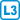## Chromatic index of planar cubic graphs

Prove that every planar cubic graph without bridges is of Vizing class I.

• #### Hint

First, color the faces, and only then derive the edge coloring from the face coloring.

• #### Solution

Let us have a planar cubic graph $$G$$ without bridges. We want to color its edges using the three colors $$a, b, c$$. According to the 4 color theorem, we first color the faces of $$G$$ using colors 1,2,3 and 4. Because the graph $$G$$ has no bridges, each edge separates different faces.

The edge gets the color according to the colors of the incident faces. The color $$a$$ corresponds to two possible pairs of face colors $$(1{,}2), (3{,}4)$$; color $$b$$ corresponds to pairs $$(1{,}3), (2{,}4)$$ and color $$c$$ to pairs $$(1{,}4), (2 , 3)$$.

Every two adjacent edges have exactly one face in common and therefore different colors.